# What if Analysis in Excel A Begginers Guide

This tutorial will explain the What if Analysis in Excel and will give a guide for beginners. Also this will define the types of what-if analysis and its uses.

## What is the what if analysis in Excel

What if Analysis is a powerful tool used to calculate complex mathematical equations with incomplete data. This function allows us to experiment and give answers to the queries with the given data and even if it is complete.

It consists of three types:

• Goal Seek
• Scenario Manager
• Data Tables

## Goal Seek

Excel What if Analysis Goal Seek is a function that helps to see potential permutations and combinations in achieving the target and goal of the consequent cell. This works opposite to formulas and functions wherein it allows you to begin with the expected result, and it computes the input value that will give the result.

## How to do a what-if analysis in Excel

To demystify this option consider the given example:

Let’s say we sample data which is the list of grades of every subject. In this case, we currently have an average grade of 78, and it needs at least an 80 to pass the class.

Fortunately, we have one final grade in Filipino that might be able to boost in the current average. Therefore we will use Goal Seek to find out what grade you need on the final to pass the class.

In the image below, you can see that the grades on the first four subjects are 55, 97, 84, and blank on Filipino. Wherein that is the goal to know the possible grade need to aim.

As you can see even if the grade in Filipino subjects is still not available we can make a formula and use the average function. So in this case the grade is weighted equally, so we will do the average of the four subjects using this formula:

=AVERAGE(I2:L2). Then once we use Goal Seek, we will show the minimum grade needed to make that grade passed.

Here are the following steps to consider:

• Step 1. Select the cell you aim to change.

So whenever using the option Goal seek of what if analysis select the cell that contains the formula or functions. In our example, we select cell M2 which uses the average function, =AVERAGE(I2:L2).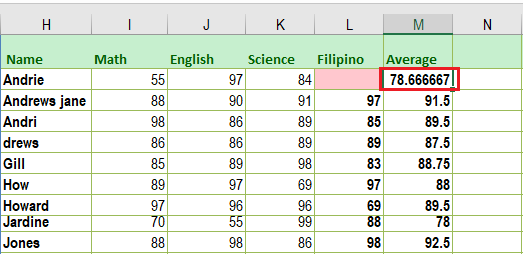• Step 2. On the Data tab, find and click the What-If Analysis command, then select Goal Seek from the drop-down menu.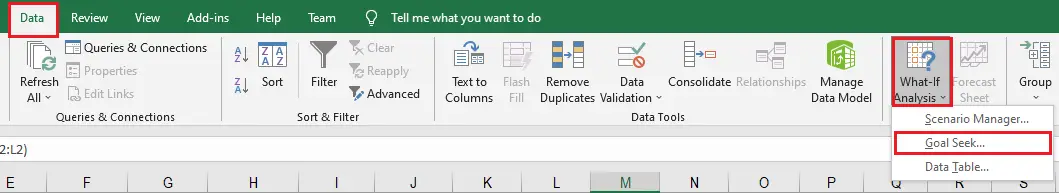• Step 3. Then a dialog box will display that contains three fields.

Set Cell is the first field which contains the desired result. In our example, cell M2 is already selected. Then the second field, To value: is the expected result. In our example, 80 is what we need to earn at least in order to pass the class.
So By changing cell: which is the third field is the cell where Goal Seek will set its answer. In our case, we’ll select cell L2 because we want to determine the grade we need to earn on the Filipino subject.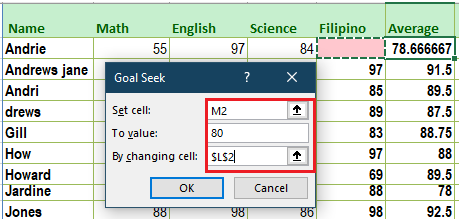• Step 4. When you’re done, click OK.

• Step 5. The dialog box will tell you if Goal Seek was able to find a solution. Click OK.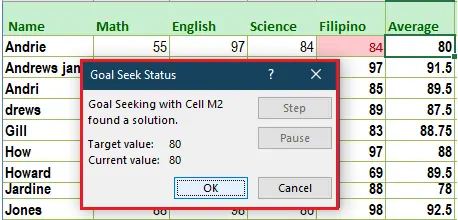• Step 6. The result will appear in the specified cell.

In our example, Goal Seek calculated that we will need a grade of at least 84 on the final to earn a passing grade.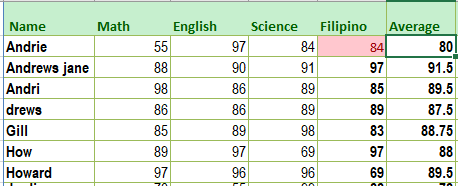## Scenario Manager

The Scenario Manager in What-if Analysis Excel is used to consume the original data and the mathematical formulas implemented on the data to recreate another scenario that inherits similarities from the previous table and generates a new table.

The scenario manager is more advanced on Goal Seek of what-if Analysis, so here are the differences between the two:

• The scenario manager can hold up to 32 changing variables at a time by creating unlimited number of possible scenarios.
• Every scenario can be saved for comparative purposes.
• It allows editing the name of Scenarios and provides a brief description.
• It only allows to change of the constant value in Scenario Manger and it should not be manually adjusted.

## How to use the Scenario Managerwhat-if analysis in Excel

To understand clearly what is scenario manager take a look at the following scenario:

• On the Data tab, in the Forecast group, click What-If Analysis.
• Click Scenario Manager.
• The Scenario Manager dialog box appears.
• Enter the scenario name (99% highest), select cell N2 for the Changing cells and click on OK.
• Enter the corresponding value of 10 and click on OK again.
• Next, add 4 other scenarios (70%, 80%, 95% and 100%).

Finally, your Scenario Manager should be consistent with the picture below:

Note: Click the Show button in order to see the result of a scenario. Then Microsoft Excel will change the value on the cell correspondingly to the result.

## What if analysis data table Excel

Data Tables of What if Analysis is an option that makes the challenging task easier, thus calculating fields and storing results in cells with just a simple drag-and-drop operation.

Additionally, Data tables allow one or two variables in a formula or replace them with as many different values as you want, then view the results in a table. This option unlike scenarios or Goal Seek is powerful because it shows multiple results at the same time.

## Conclusion

In conclusion, you already now know what IF Analysis of excel is, this time it is your time to experiment with this tool in managing your business, task, and other settings you may like. Fortunately, this tool is very useful especially when trying to know the sales or even on students which trying to earn points aiming for a passing grade or more than that.

Thank you for reading! Stay tuned to our next article.